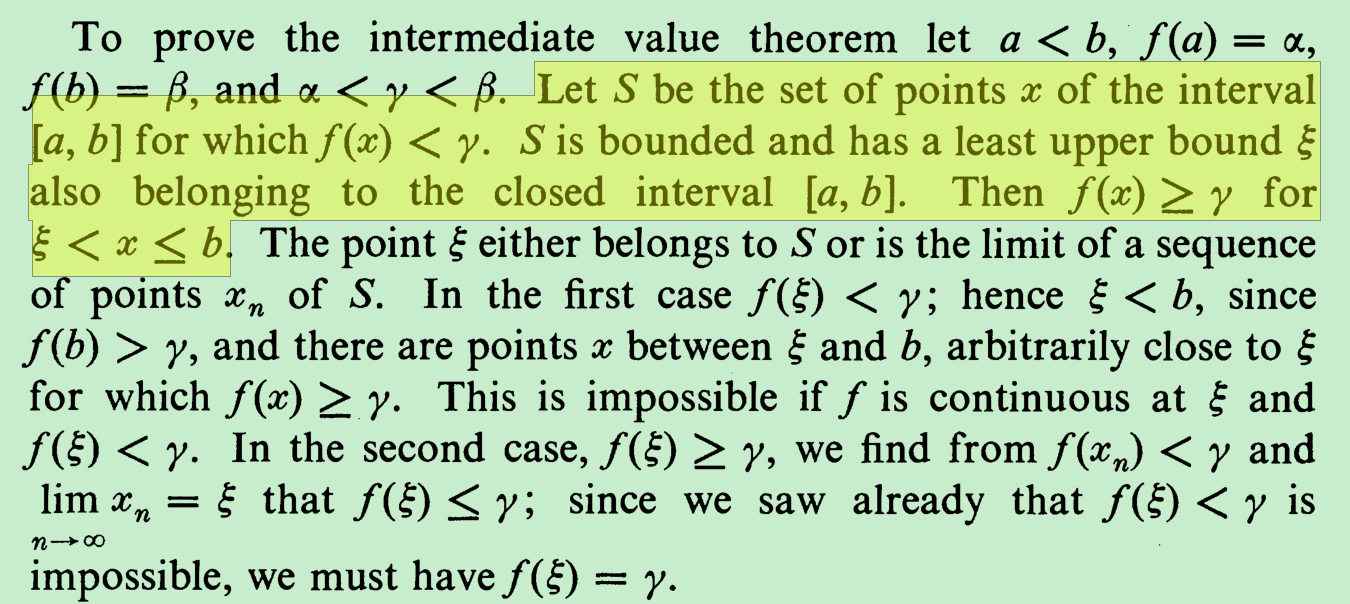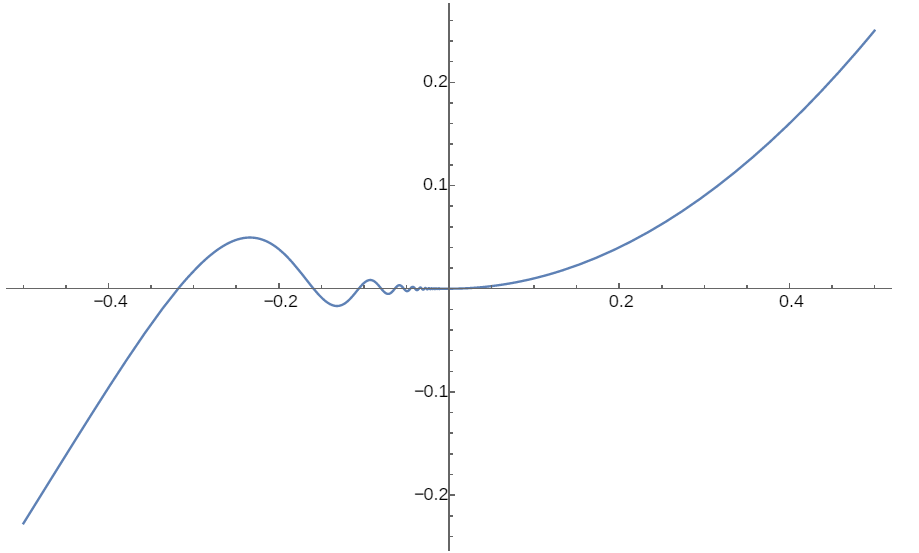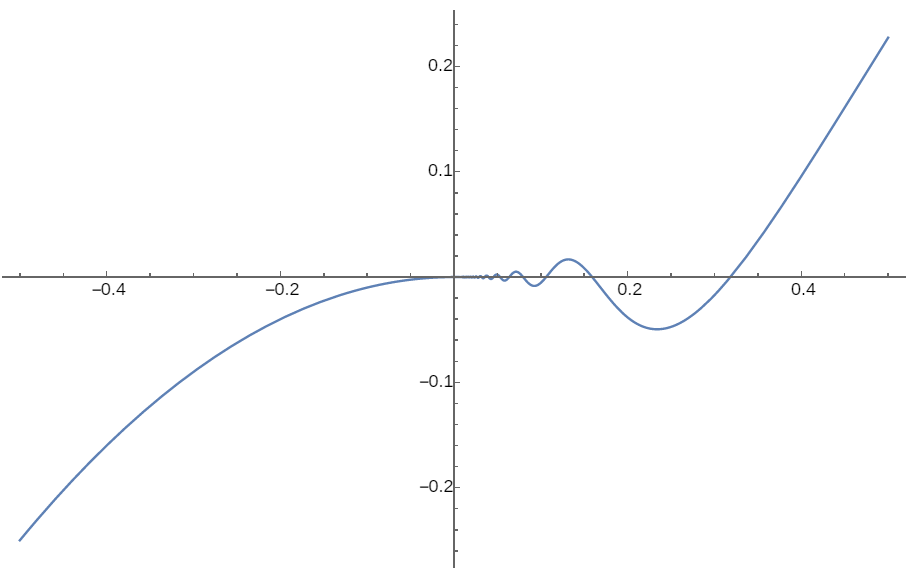# A note on trying to extend the intermediate value theorem

First, it is necessary to introduce the following definitions1,

• The function is said to be increasing at $$x_{0}$$ if for all $$x$$-values in some interval about $$x_{0}$$ it is true that when $$x_{0} < x$$ then $$y_{0} < y$$, and when $$x_{0} > x$$ then $$y_{0} > y$$.

• The function is said to be decreasing at $$x_{0}$$ if for all $$x$$-val ues in some interval about $$x_{0}$$ it is true that when $$x_{0} < x$$ then $$y_{0} > y$$, and when $$x_{0} > x$$ then $$y_{0} < y$$.

Then the definition of “a function is non-decreasing at $$x_{0}$$” is introduced by extend the definition of increasing above.

• The function is said to be non-decreasing at $$x_{0}$$ if for all $$x$$-values in some interval about $$x_{0}$$ it is true that when $$x_{0} < x$$ then $$y_{0} \leq y$$, and when $$x_{0} > x$$ then $$y_{0} \geq y$$.

The intermediate value theorem states:

If $$f$$ is a continuous function on a closed interval $$\lbrack a,b\rbrack$$, and if $$y_{0}$$ is any value strictly between $$f(a)$$ and $$f(b)$$, that is $$min\{ f(a),f(b)\} < y_{0} < max\{ f(a),f(b)\}$$, then $$y_{0} = f(c)$$ for some $$c$$ in $$(a,b)$$.

My original conjecture was to extend it to:

If $$f$$ is a continuous function on a closed interval $$\lbrack a,b\rbrack$$ with $$f(a) < f(b)$$, and if $$y_{0}$$ is any value strictly between $$f(a)$$ and $$f(b)$$, that is $$f(a) < y_{0} < f(b)$$, then $$y_{0} = f(c)$$ for some $$c$$ in $$(a,b)$$ and $$\mathbf{f}$$ is non-decreasing at the $$\mathbf{c}$$.

But it was said that the Weierstrass function, as a counterexample, is indeed nowhere monotonic, while I still couldn’t understand that. After reading the following proof for the intermediate value theorem2,I realized I could save the conjecture to:

If $$f$$ is a continuous function on a closed interval $$\lbrack a,b\rbrack$$ with $$f(a) < f(b)$$, and if $$y_{0}$$ is any value strictly between $$f(a)$$ and $$f(b)$$, that is $$f(a) < y_{0} < f(b)$$, then there is a $$c = sup(\{ x \mid f(x) < y_{0}$$ in $$(a,b)\}$$) in $$(a,b)\$$cause $$y_{0} = f(c)$$, and such that

• in every left neighborhood of $$c$$, there is always a $$x'$$ such that $$f(x') < y_{0}$$.

• for each $$x$$ in any right neighborhood of $$c,\ y_{0} \leq f(x)$$.

A similar argument could be drawn for $$f(a) > f(b)$$.

The following two functions, which are both differentiable and continuous in neighborhoods around 0, are helpful for my consideration about the question.

f(x)=\left\{\begin{aligned} x^{2} \sin \frac{1}{x}, & \text { if } x<0 \\ x^{2}, & \text { if } x \geq 0 \end{aligned}\right.f(x)=\left\{\begin{aligned} -x^{2}, & \text { if } x \leq 0 \\ x^{2} \sin \frac{1}{x}, & \text { if } x>0 \end{aligned}\right.1. Calculus: An Intuitive and Physical Approach, Second Edition, Morris Kline, Chapter 8，Section 3↩︎

2. Introduction to Calculus and Analysis Volume I, Reprint of the 1989 edition, Richard Courant, Fritz John, p101↩︎